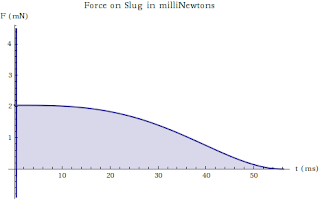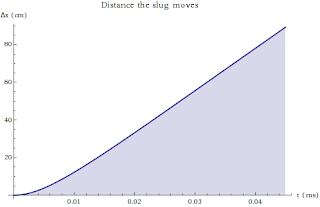# Railguns

### So me and Jesse got to thinking today about Railguns. Every year in Physics 213 a common homework problem is a rather simple model of a railgun. We tried to think about a more realistic model. We simulated a rail connected to a voltage source with a limiting resistor, moving under its own magnetic field with a back-emf.Short answer: Doesn't work very well if you limit the current, but works great if you dump a MA or so down the wire.

See the full solution after the jump.

#### Setup

Imagine you have two closely spaced conducting rails which you connect to a voltage source. Laid across these two rails is another piece of conductor. The first thing to notice is that we expect the conductor, if we assume it can slide along the rails without friction, will be compelled to move because of the magnetic force caused by the magnetic field from the rails acting on the current through the segment. Lets be quantitative, our voltage source provides and emf V. The rails are seperated by some distance L. The initial position of the slug (as it will be termed) is $x_0$ We are interested in the motion of the slug. It will feel a force $$F = I \int B \cdot dl$$ The current being the current that flows through the circuit. The important contributions to the current is the emf of the source, the resistivity of the rails and slug, and the back emf induced by the motion of the slug. I.e. our equation for the circuit takes the form $$V - I\lambda( L + 2x ) - \frac{d}{dt} \int B dA = 0$$ We need to approximate the magnetic field acting on the slug. We will treat this magnetic field as originating wholly from the rails, which we will treat as semi-infinite wires. This approximation should be good in the limit that $L \gg x_0$ The magnetic field of a semi-infinite wire is $$B = \frac{\mu_0}{4\pi} \frac{I}{r}$$ so our magnetic field acting on the slug, if we treat $z=0$ to be at the bottom rail and $z=L$ to be at the top is given by $$B = \frac{\mu_0}{4\pi}I \left( \frac{1}{z} + \frac{1}{L-z} \right)$$ We can calculate the influence of this field on the slug. Of course if we integrate this field from 0 to L it will blow up, so we need to set some cutoff, $R$ which will stand in for the fact that our wires have some finite thickness. We proceed: $$\int B\ dl = \frac{\mu_0}{4\pi} I \int_{R}^{1-R} \frac{1}{z} + \frac{1}{L-z} \ dz = \frac{\mu_0}{4\pi} I \int_{\epsilon}^{1-\epsilon} \frac{1}{\xi} + \frac{1}{1-\xi} \ d\xi$$ where I have non dimensionalized the integral with: $$\epsilon = R/L$$ We obtain $$\int B\ dl = \frac{\mu_0}{4\pi} \left[ 2 \log \left( 1/\epsilon - 1 \right) \right] I \equiv \kappa I$$ This gives us the force on our slug $$F = \kappa I^2$$ as well as an approximation for the magnetic flux if we assume that this field approximation works for the length of the rails (up to the slug) $$\int B \ dA = \kappa I x$$ So that we can gather our circuit equation $$V - I\lambda( L + 2x ) - \kappa \frac{d}{dt}(I x) = 0$$ and the equation of motion for our slug $$m \ddot x = \kappa I^2$$ Lets nondimensionalize these equations with $$x = L \chi$$ $$x_0 = f L$$ $$I = \frac{V}{r + \lambda L (1+ 2f)} \Phi \equiv I_0 \Phi$$ marking the initial current that will flow through the slug. $$t = \frac{ \kappa}{2 \lambda } \tau$$ giving a characteristic time. Putting it all together, our differential equations become much simpler. $$f - \chi - \frac{d}{d\tau} \left( \Phi \chi \right) = 0$$ $$\frac{d^2 \chi}{d\tau^2} = \beta \Phi^2$$ $$\beta = \frac{ \kappa^3 I_0^2 }{ 4 m L \lambda^2 }$$ So that our system is specified completely by the dimensionless constants f, β, f having something to say about the initial geometry of our system, and β speaking to the relative mass of the slug.

#### Analysis

These coupled nonlinear differential equations are not easily solved, but by taking the time to nondimensionalize them, we can integrate them numerically. Lets suppose our rails are separated by 1 cm, and our f is taken to be 10 or so (remember the larger f, the better our approximation for the field). And for our material properties, lets take gauge 0 copper wire, i.e. I will use its radius, mass density, and conductivity at room temperature. Lets make the voltage supply be a kilovolt (non unreasonable), and lets choose the limiting resistor in order to keep the current in the gauge 0 wire under its specification: 170 amps. Doing all of this, we have $$\beta \approx 5.4 \times 10^{-7}$$ $$f = 10$$ and for interest our characteristic time is 112 microseconds or so, the mass of our slug is about 5 grams. Our average magnetic field is about 0.07 gauss/amp between the rails, so that our starting field is about 12 gauss.#### Conclusions

So it looks like our Railgun doesn't do very much at all. In fact, its quite paltry, achieving very puny speeds. What went wrong? Well, it seems the biggest problem comes from limiting the maximum current that can go down our wire. The truth is that if we want to really project some stuff forward, we're either going to need much higher currents, or some external field. You'll notice that most of the action takes place over a very short time scale, so it could be that we could violate the rated currents of our wire. If that's the case, setting our failsafe resistor to zero, I'll just show two more graphs, that of the velocity and position of the slug:This is starting to look a lot more like a Railgun, unfortunately, it would draw a current of 15 MA to start! So the real question becomes, how much current can you dump into a copper wire in 0.1 ms or so. 15 MA is a lot and would certainly melt the wire, but would it melt the wire in such a short time? If not, then it looks like we could get some real kick out of our model. In fact, we know that railguns with some kick can be made. According to Wikipedia, the US Navy has a railgun that can accelerate a 3.2 kg projectile at 7 times the speed of sound (\~2.3 km/s). Playing with my code, if I put in by hand a projectile mass of 3.2 kg, and reduce f to 1 (meaning we get a little more kick but our approximation of the field starts to really break down), I can get a final speed of about 2 km/s, which seems to be in relatively good agreement, but this only occurs if the current is \~100 MA for about 30 microseconds. Moral of the story, if you want to build a railgun, you need to be willing to melt some copper. Attached is a Mathematica notebook used for the computation: here.